HomeenProblem of determining the average atomic mass from the isotopic abundances

# Problem of determining the average atomic mass from the isotopic abundances

Atoms are the fundamental units that make up the different chemical elements that, in turn, are part of matter. While it is true that two atoms of the same element have the same number of protons and electrons and share essentially the same chemical properties, not all atoms of the same element are the same. This is due to the existence of isotopes, which are nothing more than atoms of the same element, but have different mass numbers.

But if in a pure sample of any element there is actually a mixture of atoms with the same properties but different masses, why does the periodic table only show one atomic mass for each element?

The answer is that the periodic table does not actually show the mass of one atom of each element, but instead shows the average mass of all the atoms present in a natural sample of that element.

## Atomic Mass vs. Average Atomic Mass

As its name indicates, the atomic mass corresponds to the mass of an individual atom. That is, it is the mass corresponding to an atom of a particular isotope of a chemical element. As expected, it is an extremely small mass; so small, in fact, that it is expressed in special units of mass called the atomic mass unit, or amu .

For its part, the average atomic mass represents, as previously stated, the average mass of all the atoms present in a natural sample of an element. This mass is calculated as the average mass of all naturally occurring isotopes of an element, weighted by their natural relative isotopic abundance. That is to say:Where MA i represents the atomic mass of the natural isotope i, and %A i represents the relative abundance as a percentage of said isotope. For the application of this equation, all the masses and abundances of all the natural isotopes of an element are required.

Isotopes that are unstable and therefore decay radioactively over time, transforming into different atoms, are not included in the sum.

The following solved problems will serve to exemplify the use of this formula in determining the average atomic mass of an element.

## Example 1: Determination of the average atomic mass from isotopic abundances

### statement

Selenium is a nonmetal that has six stable isotopes, all with isotopic abundances less than 50%. The most abundant isotope is selenium-80, which accounts for almost half of all the selenium atoms in a natural sample of the element. The following table lists each of these isotopes along with their relative abundance and atomic mass as determined using the mass spectrometry technique. Determine the average atomic mass of selenium.

Isotope Atomic mass (amu) % Abundance 74 It 73.922477 0.89 76 It 75.919214 9.37 77 will 76.919915 7.63 78 It 77.917310 23.77 80 It 79.916522 49.61 82 It 81.916700 8.73

### Solution

This type of problem consists of the direct application of the previous equation. As you can see we have all the necessary data for the determination of the atomic weight or average atomic mass.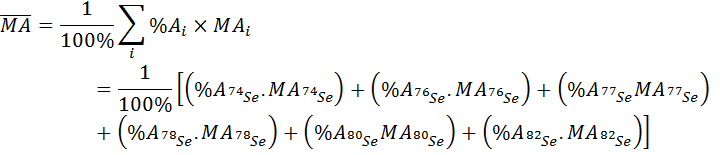Therefore, the average atomic mass of selenium is 78.96 amu.

## Example 2: Determination of the abundance of an isotope from the average atomic mass

### statement

Iron is an element found in many meteorites, and the ratio in which its four stable isotopes are found provides important information about the meteorite’s provenance and age. A sample from the YuB-2021 meteorite was analyzed and found that the iron present in it has an average atomic mass of 55.8074 uma, slightly lower than the average atomic mass of terrestrial iron, which is 55.845 uma. The reason is presumed to be a higher proportion of the lighter isotope iron-54 (which on planet Earth has an abundance of 5.845%); however, neither the abundance of this isotope nor that of the less abundant iron-58 could be determined with good accuracy. Using the data presented below, determine the two missing isotopic abundances,

Isotope Atomic mass (amu) % Abundance 54 faith 53.9396105 ? 56 faith 55.9349375 89.9373 57 faith 56.9353940 2.0770 58 faith 57.9332756 ?

### Solution

Unlike the previous problem, in this case the average atomic mass and abundances of two of the four iron isotopes are known in advance. The average atomic mass formula will not suffice to determine the abundance of the two missing isotopes, since such an equation would have two unknowns.

In order to solve the problem, we must, then, find some other mathematical relationship between the variables involved, in order to establish a system of equations that allows us to obtain both unknowns. In this case, the second equation consists of the sum of the abundances of all the isotopes, which must be 100%.

So we establish the following system of equations: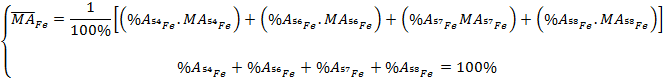This system of equations can be easily solved by means of the following steps:

1. The first equation is linearized by multiplying both sides by 100.• The second is solved for any of the two unknowns (%A 54Fe or %A 58Fe ).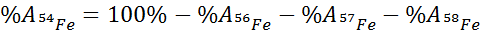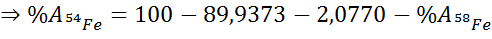• Substitute the expression obtained in the previous step into the first equation.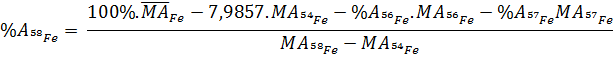• The first equation is solved for the second unknown and its value is calculated.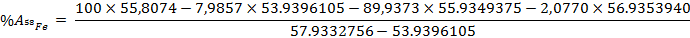• The value of the unknown calculated in the previous step is substituted in the expression of the first unknown and its value is calculated: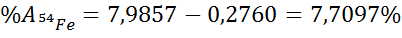As can be seen, the abundance of iron isotope 54 on the asteroid was found to be 7.7097%, which is considerably higher than the 5.845% abundance of this isotope on Earth.

## References

Chang, R. (2021). Chemistry (Ninth ed.). McGraw-Hill.

Garcia, S.A. (nd). Isotopes Table . University of Antioquia. http://sergioandresgarcia.com/pucmm/fis202/4.TI.Tabla%20de%20isotopos%20naturales%20y%20abundancia.pdf

Gaviria, JM (2013, August 9). Calculation of the relative abundances of carbon isotopes . TRIPLELINK. https://triplenlace.com/2013/08/09/calculo-de-las-abundancias-relativas-de-los-isotopos-del-carbono/